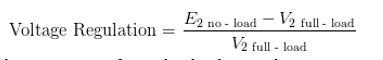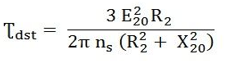Engineering Jobs   »   uprvunl je electrical quiz

# UPRVUNL’21 EE: Daily Practices Quiz 29-July-2021

Each question carries 1 mark.
Negative marking: 1/4 mark
Total Questions: 06
Time: 08 min.

Q1. The voltage regulation of a transformer at full load 0.9 power factor lagging is 5%. For a full load at 0.9 power factor leading, it will:
(a) Remain the same
(b) Become negative
(c) Reduce and may even become negative
(d) Increase

Q2. Schering bridge is used to measure
(a) Dielectric loss
(b) The inductance
(c) Low resistance
(d) Mutual Inductance

Q3. If a resistance is inserted in the rotor circuit of a slip ring induction motor, then compared to direct line starting:
(a) Both the starting current and torque reduce
(b) Both the starting current and torque increase
(c) The starting current reduces but starting torque increases
(d) The starting current reduces but starting torque

Q4. The colour of the earth wire is generally:
(a) Red
(b) Blue
(c) Black
(d) Green

Q5. Strength of the electric field is ___________
(a) Directly proportional to the force applied
(b) Inversely proportional to the force applied
(c) Directly proportional to the square of the force applied
(d) Inversely proportional to the square of the force applied

Q6. Ferranti effect can be compensated by which of the following
(a) Shunt capacitor
(b) Shunt reactor
(c) Series capacitor
(d) Both a and b

SOLUTIONS
S1. Ans.(c)
Sol.• For lagging power factor loads, the regulation is positive because for lagging power factor load the secondary voltage decreases with increase in the load current (when the transformer is loaded).
• For leading power factor loads, the regulation is negative (raise in voltage as load current increases).
Hence for leading power factor voltage regulation decrease and may even become negative

S2. Ans.(a)
Sol. Schering bridge is used to measure dielectric loss. It is an AC bridge circuit that is used for measuring the insulating properties of electrical cables and equipment. It has the advantage that the balance equation is independent of frequency.
• Maxwell bridge is used to measure the value of unknown inductance.
• Heaviside bridge is used to measured mutual inductance in terms of a known self-inductance.
• Kelvin bridge is used to measure the value of low resistance.

S3. Ans.(c)
Sol. Starting torque is directly proportional to the rotor resistance of an induction motor.In slip ring induction motor the ends of the rotor windings are externally connected by a variable rheostat. So more the resistance, more the torque. When we add resistance to the rotor, the torque is high, the slip is high and the current is reduced. Therefore, slip ring induction motor is used for providing high starting torque.

S4. Ans.(d)
Sol. The colour of earth wire is generally green in colour. Green wire stands for grounding / earthing in an electric circuit.
The colour coding of wires is:
• Green for earth
• Red for live
• Black for neutral.

S5. Ans.(a)
Sol. Electric field intensity is the force per unit charge; hence it is directly proportional to the force applied.

S6. Ans.(b)
Sol. Ferranti effect occurs in case of no load or light load condition of a medium and long transmission lines due to capacitance effect of the transmission line.
Shunt reactor will reduce the effect of capacitance. Therefore, Ferranti effect will be reduced.

Sharing is caring!